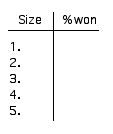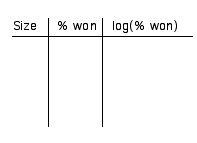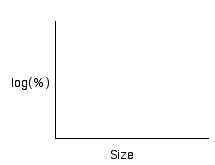# Disk Game

The game is played by tossing a disk on tiles 9 inches square. To win, you must toss the disc so it lands on a crack. If the winner gets \$2, what would be a fair price to play the game? It depends more on the size of the disk than the skill of the player.

Answer the questions on page 178.

# Challenge Questions:

1. Using 9 inch tiles, find the win/loss proportion for 5 different sizes of disk (all under 4 inches), and record the size and percentage of wins.2. Make a scatterplot of your data.3. Find the linear regression line. What is the equation?
4. Use the equation to predict the percentage of wins for 5, 6, and 7 inch disks.5. Use the simulation to see if the data match your predictions.
6. Did they match?
7. Try a non-linear model (make a linear regresson line with the log(% of wins) vs. size rather than % of wins vs. size like we did last week in class... see pages 180-181, 185.8. What is the the equation for the logarythmic model (see p. 185)?
9. Use this equation to predict 5, 6, and 7 inch disks. Is it a better estimate than the first equation you used?
10. If the disk is 3" and the tile is 9" what would be a fair jackpot if it costs \$1 to play?
11. What would be a fair size disk (50% wins) for 4 inch tiles?
12. What would be a fair size disk (50% wins) for 6 inch tiles?
13. What would be a fair size disk (50% wins) for 9 inch tiles?
14. What would be a fair size disk (50% wins) for 12 inch tiles?
15. What would be a fair size disk (50% wins) for 16 inch tiles?
16. Is there a pattern? Make a scatterplot/regression line to predict the fair size disk for a 18, 20, and 24 inch grid.

This simulation was written by Fr. Chris Thiel, OFMCap (cthiel@sfhs.net). If you want to start learning how to program, take a look at the Java source code.